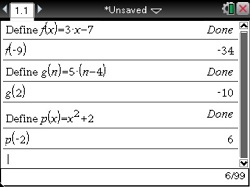•##### Download

•• ##### Device
• TI-Nspire™
• TI-Nspire™ CAS

2.1

• ##### Report an Issue

Algebra 1: Computing with Mathematical Formulas
by Brendan Kelly#### Objectives

• Evaluate any algebraic expression for real number values of its variables
• Use technology to verify the evaluation of any algebraic expression for real values of its variables

#### About the Lesson

Students are sometimes introduced to algebra and algebraic operations in a formal way, using rules and procedures but without an appreciation of why such abstract formulations are needed. Having students evaluate formulas for given values of a variable is an excellent way to acquaint them with the idea that a variable is a "place-holder" to which various values can be assigned. A formula is then understood as an "input-output" machine that takes any value assigned to a variable, performs operations on this value, and then yields an output.

In this activity, we introduce the formula relating the time needed to cook a turkey to its mass. Even if you are conducting this activity long before Thanksgiving, this formula will be of interest to your class of hungry teenagers who will readily gobble it up. Certainly, the importance of understanding the units used in a formula will be clear from the cartoon.

©Brendan Kelly Publishing, Inc. Reprinted with permission. www.brendankellypublishing.com